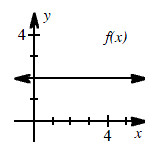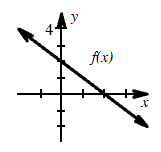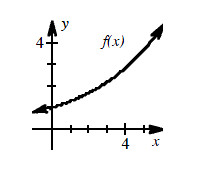### Home > CALC > Chapter 3 > Lesson 3.3.2 > Problem3-108

3-108.

For each graph below:

1. Trace $f(x)$ on your paper and write a slope statement for $f(x)$.

Remember, slope statements start on the left and end on the right. They describe how the slope changes.

2. Sketch the graph of $f^\prime(x)$ using a different color.

1.1.1.